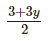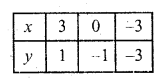• +91 9971497814
• info@interviewmaterial.com

# RD Chapter 3- Pair of Linear Equations in Two Variables Ex-3.2 Interview Questions Answers

### Related Subjects

Question 1 : Solve the following systems of equations graphically :
x + y = 3
2x + 5y = 12 (C.B.S.E. 1997)

x + y = 3
=> x = 3 – y
Substituting some different values of y, we get the corresponding values of x as shown below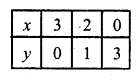Now plot the points on the graph and join them 2x + 5y = 12
2x = 12 – 5y
⇒ x =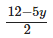Substituting some different values of y, we get the corresponding values of x as shown below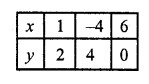Now plot the points on the graph and join them we see that these two lines intersect each other at (1, 2)
x = 1, y = 2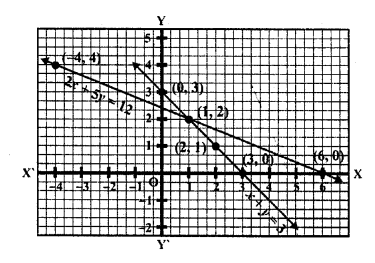Question 2 :
x – 2y = 5
2x + 3y = 10 (C.B.S.E. 1997)

x – 2y = 5 => x = 5 + 2y
Substituting some different values of y, we get the corresponding values of x as shown below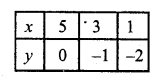Now plot the points are the graph and join them
2x + 3y = 10 => 2x = 10 – 3y
⇒ x =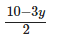Substituting some different values of y We get the corresponding values of x as shown below :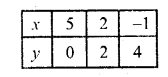Now plot the points on the graph and join them we see that these two lines intersect each other at (5, 0)
x = 5, y = 0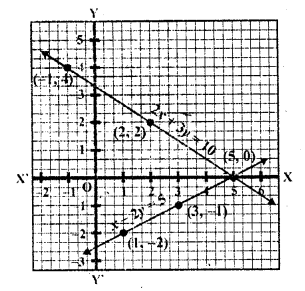Question 3 :
3x + y + 1 = 0
2x – 3y + 8 = 0 (C.B.S.E. 1996)

3x + y + 1 = 0
y = -3x – 1
Substituting the values of x, we get the corresponding values of y, as shown below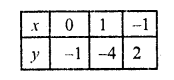Now plot the points on the graph and join them
2x – 3y + 8 = 0
⇒ 2x = 3y – 8
⇒ x =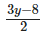Substituting some different values of y, we get the corresponding values of x as shown below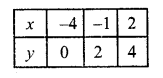Now plot the points on the graph and join then we see that these two lines intersect, each other at (-1, -2)
x = -1, y = 2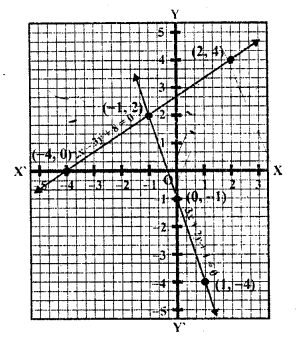Question 4 :
2x + y – 3 = 0
2x – 3y – 7 = 0 (C.B.S.E. 1996)

2x + y – 3 = 0 => y = -2x + 3
Substituting some different values of x, we get the corresponding values of y as shown below: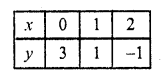Now plot the points and join them 2x – 3y – 7 = 0
2x = 3y +7
x =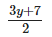Substituting some different values of y, we get corresponding values of x as shown below: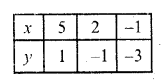Now plot the points on the graph and join them we see that these two lines intersect each other at (2, -1)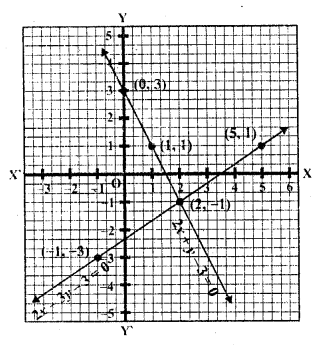Question 5 :
x + y = 6
x – y = 2 (C.B.S.E. 1994)

x + y = 6 => x = 6 – y
Substituting some different values of y, we get the corresponding values of x as shown under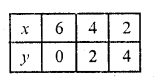Now plot the points on the graph and join them
x – y = 2 ⇒ x = 2 + y
Substituting some different values of y, we get the corresponding values of x as shown below: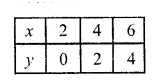Now plot the points on the graph and join them
We see that there two lines intersect each other at (4, 2)
x = 4, y = 2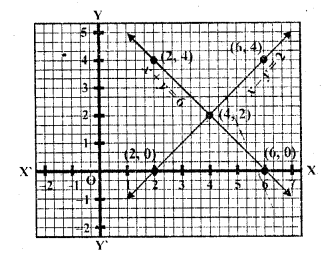Question 6 :
x – 2y = 6
3x – 6y = 0 (C.B.S.E. 1995)

x – 2y = 6
x = 6 + 2 y
Substituting some different values ofy, we get the corresponding values of x as shown below: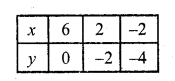Now plot the points and join them
3x – 6y = 0 ⇒ 3x = 6y ⇒ x = 2y
Substituting some different value of y, we get corresponding the values of x as shown below: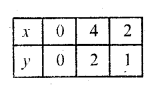plot the points on the graph and join them We see that these two lines intersect each other at no point
The lines are parallel
There is no solution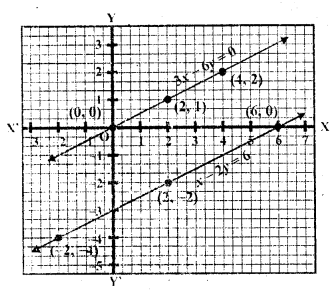Question 7 :
x + y = 4
2x – 3y = 3 (C.B.S.E. 1995)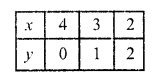⇒ x =